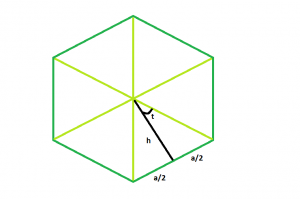# Area of a n-sided regular polygon with given side length

Given a regular polygon of N sides with side length a. The task is to find the area of the polygon.

Examples:

```Input : N = 6, a = 9
Output : 210.444

Input : N = 7, a = 8
Output : 232.571
```

## Recommended: Please try your approach on {IDE} first, before moving on to the solution.Approach: In the figure above, we see the polygon can be divided into N equal triangles. Looking into one of the triangles, we see that the whole angle at the center can be divided into = 360/N

So, angle t = 180/n
Now, tan(t) = a/2*h

So, h = a/(2*tan(t))

Area of each triangle = (base * height)/2 = a * a/(4*tan(t))
So, area of the polygon,

```A = n * (area of one triangle) = a2 * n/(4tan t)
```

Below is the implementation of the above approach:

## C++

 `// C++ Program to find the area of a regular ` `// polygon with given side length ` ` `  `#include ` `using` `namespace` `std; ` ` `  `// Function to find the area ` `// of a regular polygon ` `float` `polyarea(``float` `n, ``float` `a) ` `{ ` `    ``// Side and side length cannot be negative ` `    ``if` `(a < 0 && n < 0) ` `        ``return` `-1; ` ` `  `    ``// Area ` `    ``// degree converted to radians ` `    ``float` `A = (a * a * n) / (4 * ``tan``((180 / n) * 3.14159 / 180)); ` ` `  `    ``return` `A; ` `} ` ` `  `// Driver code ` `int` `main() ` `{ ` `    ``float` `a = 9, n = 6; ` ` `  `    ``cout << polyarea(n, a) << endl; ` ` `  `    ``return` `0; ` `} `

## Java

 `// Java  Program to find the area of a regular ` `// polygon with given side length ` ` `  `import` `java.io.*; ` ` `  `class` `GFG { ` `   `  ` `  `// Function to find the area ` `// of a regular polygon ` `static` `float` `polyarea(``float` `n, ``float` `a) ` `{ ` `    ``// Side and side length cannot be negative ` `    ``if` `(a < ``0` `&& n < ``0``) ` `        ``return` `-``1``; ` ` `  `    ``// Area ` `    ``// degree converted to radians ` `    ``float` `A = (a * a * n) /(``float``) (``4` `* Math.tan((``180` `/ n) * ``3.14159` `/ ``180``)); ` ` `  `    ``return` `A; ` `} ` ` `  `// Driver code ` ` `  `    ``public` `static` `void` `main (String[] args) { ` `    ``float` `a = ``9``, n = ``6``; ` ` `  `    ``System.out.println( polyarea(n, a)); ` `    ``} ` `} ` `// This code is contributed by inder_verma.. `

## Python3

 `# Python 3 Program to find the area  ` `# of a regular polygon with given ` `# side length ` `from` `math ``import` `tan ` ` `  `# Function to find the area of a  ` `# regular polygon ` `def` `polyarea(n, a): ` `     `  `    ``# Side and side length cannot  ` `    ``# be negative ` `    ``if` `(a < ``0` `and` `n < ``0``): ` `        ``return` `-``1` ` `  `    ``# Area degree converted to radians ` `    ``A ``=` `(a ``*` `a ``*` `n) ``/` `(``4` `*` `tan((``180` `/` `n) ``*` `                      ``3.14159` `/` `180``)) ` ` `  `    ``return` `A ` ` `  `# Driver code ` `if` `__name__ ``=``=` `'__main__'``: ` `    ``a ``=` `9` `    ``n ``=` `6` ` `  `    ``print``(``'{0:.6}'``.``format``(polyarea(n, a))) ` ` `  `# This code is contributed by ` `# Shashank_sharma `

## C#

 `// C# Program to find the area of a regular ` `// polygon with given side length ` `using` `System; ` ` `  `class` `GFG  ` `{ ` ` `  `// Function to find the area ` `// of a regular polygon ` `static` `float` `polyarea(``float` `n, ``float` `a) ` `{ ` `    ``// Side and side length cannot be negative ` `    ``if` `(a < 0 && n < 0) ` `        ``return` `-1; ` ` `  `    ``// Area ` `    ``// degree converted to radians ` `    ``float` `A = (a * a * n) / (``float``)(4 * Math.Tan((180 / n) * ` `                                           ``3.14159 / 180)); ` ` `  `    ``return` `A; ` `} ` ` `  `// Driver code ` `public` `static` `void` `Main () ` `{ ` `    ``float` `a = 9, n = 6; ` `     `  `    ``Console.WriteLine(polyarea(n, a)); ` `} ` `} ` ` `  `// This code is contributed ` `// by Akanksha Rai `

## PHP

 ` `

Output:

```210.444
```

My Personal Notes arrow_drop_upCheck out this Author's contributed articles.

If you like GeeksforGeeks and would like to contribute, you can also write an article using contribute.geeksforgeeks.org or mail your article to contribute@geeksforgeeks.org. See your article appearing on the GeeksforGeeks main page and help other Geeks.

Please Improve this article if you find anything incorrect by clicking on the "Improve Article" button below.